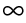Manipulating numeric data
Numeric data is probably the most commonly manipulated type of data. Expressions can perform a basic operation, such as multiplying a price field by a quantity field to calculate an extended price, or more complex calculations, such as a financial calculation that returns the depreciation value of an asset. Use Aggregation Builder to calculate aggregate information, such as totals, averages, medians, modes, and so on, as discussed in Aggregating data.
Both JavaScript and BIRT provide a wide range of functions for manipulating numeric data. In the expression builder, look under Native JavaScript Functions—Number and Math, and under BIRT Functions—BirtMath and Finance. The following sections describe common number-manipulation tasks and provide examples of expressions.
Computing values from multiple numeric fields
If a report primarily displays values from numeric fields, it most likely contains computed values as well. An invoice, for example, typically shows the following computed values:Extended prices that display the product of unit price  quantity for each line itemSales tax total that displays the product of extended prices  tax rateInvoice total that displays the sum of extended prices + shipping + sales tax
Order of operations
When a calculation involves more than two numbers and different operators, remember the order of operations, which is the order in which operators are evaluated. Consider the following math expression:
55 + 50 + 45 2
If you performed each operation from left to right in the following steps:
55 + 50 = 105
105 + 45 = 150
150 2 = 300
If you specify the math expression in a data element, BIRT Report Designer returns 195, which is the correct answer. The difference in answers lies in the order of operations. This concept is one that you might remember from math class. Multiplication and division are evaluated first from left to right across the expression. Then, addition and subtraction are evaluated from left to right across the expression. Using the previous example, the expression is evaluated as follows:
45 2 = 90
55 + 50 = 105
105 + 90 = 195
To perform the addition before the multiplication, enclose the addition part within parentheses, as follows:
(55 + 50 + 45) 2
The following list describes examples of expressions that compute values from multiple numeric fields:The following expression calculates a total price after deducting a discount and adding an 8% tax that applies to the discounted price:
(row["extendedPrice"] - row["discount"]) + (row["extendedPrice"] - row["discount"]) * 0.08The following expression calculates an invoice total, which includes the total of all extended prices, an 8% sales tax, and a 10% shipping and handling charge:
row["salesTotal"] + (row["salesTotal"] * 0.08) + (row["salesTotal"] * 0.10)The following expression calculates a gain or loss in percent:
(row["salePrice"] - row["unitPrice"])/row["unitPrice"] * 100
Division by zero
If you divide the value of one numeric field by another and the denominator value is 0, the result is infinity ().
For example, if the following expression:
row["total"]/row["quantity"]
evaluates to:
150/0
the data element that contains the expression displays.
The return value is infinity because dividing a number by zero is an operation that has no answer. Mathematicians consider this operation undefined, illegal, or indeterminate.
If you do not want the infinity symbol to appear in the report, you can replace it with a string value, such as Undefined, or replace it with an empty string ("") to display nothing. The infinity symbol is a numeric value; therefore, you must convert it to a string before replacing it with a different string.
The following expression replaceswith Undefined:
// Convert number to a string
x = row["total"]/row["quantity"] + ""
// Replacewith the word Undefined
x.replace("Infinity", "Undefined")
Alternatively, use the BirtMath function, safeDivide( ). The safeDivide( ) function takes three arguments: the number to divide, the divisor, and the value to return if the result of the division is infinity. The following expression uses safeDivide( ) to divide row["total"] by row["quantity"] and to return Undefined for an infinity value:
BirtMath.safeDivide(row["total"], row["quantity"], "Undefined")
Converting a number to a string
Convert a number to a string using one of the following techniques:Use the JavaScript toString( ) function.Add an empty string ("") to the number.
The following expressions yield the same result. If the value of orderID is 1000, both expressions return 10005.
row["orderID"].toString() + 5
row["orderID"] + "" + 5
Any time you combine a literal string with a number, JavaScript converts the number to a string. Be aware of this fact, especially if you want to also manipulate the number mathematically. For example, the following expression changes orderID to a string:
"Order ID: " + row["orderID"]
To perform a calculation and add a literal string, do them in separate steps. Perform the calculation first, then append the string, as shown in the following example:
orderIDvar = row["orderID"] + 10;
"Order ID: " + orderIDvar;
If the value of orderID is 1000, the expression returns:
Order ID: 1010